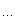# sunkehappy

## 杭电1398#include <iostream>using namespace std;const int lmax=10000;//c1是用来存放展开式的系数的，而c2则是用来计算时保存的，//他是用下标来控制每一项的位置，比如 c2 就是 x^3 的系数。//用c1保存，然后在计算时用c2来保存变化的值。int c1[lmax+1],c2[lmax+1];int main(){int n, i, j, k ;// 计算的方法还是模拟手动运算，一个括号一个括号的计算，// 从前往后while ( cin>>n ){//对于 1+x+x^2+x^3+他们所有的系数都是 1// 而 c2全部被初始化为0是因为以后要用到 c2[i] += x ;for ( i=0; i<=n; i++ ){c1[i]
=1;c2[i]
=0;}//第一层循环是一共有 n 个小括号，而刚才已经算过一个了//所以是从2 到 nfor (i=2; i<=n; i++){// 第二层循环是把每一个小括号里面的每一项，都要与前一个//小括号里面的每一项计算。for ( j=0; j<=n; j++ )//第三层小括号是要控制每一项里面 X 增加的比例// 这就是为什么要用 k+= i ;for ( k=0; k+j<=n; k+=i ){// 合并同类项，他们的系数要加在一起，所以是加法，呵呵。// 刚开始看的时候就卡在这里了。c2[j+k] += c1[j];}// 刷新一下数据，继续下一次计算，就是下一个括号里面的每一项。for ( j=0; j<=n; j++ ){c1[j]
= c2[j] ;c2[j]
= 0 ;}}cout
<<c1[n]<<endl;}return 0;}

posted on 2009-04-29 10:47 祝你好运！ 阅读(142) 评论(0)  编辑 收藏 引用